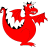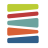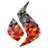22-26 March 2021

# Implementation of p-multigrid and approximate fast diagonalization methods in Firedrake

Pablo Brubeck (University of Oxford, 🇬🇧)
Patrick Farrell (University of Oxford, 🇬🇧)
Monday session 2 (Zoom) (15:00–16:30 GMT)
10.6084/m9.figshare.14495202

For problems with smooth solutions, high-order methods have very good convergence properties, and in some cases they do not exhibit locking phenomena found in low-order methods. Moreover, due to data-locality and high arithmetic intensity, they are better suited to make efficient use of modern parallel hardware architectures. Unfortunately, the conditioning of the Galerkin matrices is severely affected by $$p$$, the polynomial degree of the approximation. In order to obtain practical iterative solvers, we require good preconditioners.

In the $$p$$-variant of multigrid, the problem is often coarsened by rediscretizing on the same mesh with a lower $$p$$. We implement a general $$p$$-multigrid ($$p$$-MG) method that can deal with general finite elements and custom coarsening schedules inFiredrake usingPETSc. As relaxation, we employ a novel combination of an approximate fast diagonalization method and subspace correction. The scheme is essentially point-block Jacobi in the space of eigenfunctions of a separable approximation to the local stiffness matrix of each cell. The relaxation depends on the tensor-product structure of quadrilateral and hexahedral elements, in a similar manner to sum factorisation.

We employ this relaxation method in two algorithms: a $$p$$-MG preconditioner and a full approximation scheme nonlinear solver. We demonstrate how to combine these two efficiently in a nested iteration with a cascadic outer cycle and inner V-cycles. All available solvers, including geometric and algebraic multigrid, may be employed for the $$p$$-coarse level. The associated computational costs are $$O(p^d)$$ to approximate the local stiffness matrix in $$d$$ dimensions, and $$O(p^{d+1})$$ to apply or update the relaxation, while memory requirements are kept at $$O(p^d)$$. We present nonlinear examples such as the $$p$$-Laplacian and incompressible hyperelasticity.